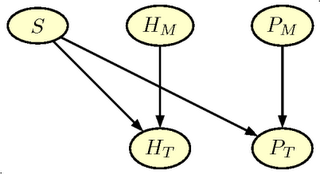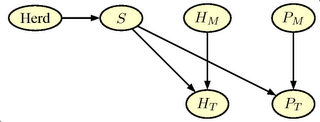## Wednesday, December 06, 2006

### Enhanced pregnancy testing

The value of pregnancy testing is often over-estimated, because the pregnancy test is not the only test used. After three weeks many of the non-pregnant sows will return to oestrus. Oestrus signs are a very specific indicator of non-pregnancy. If this Oestrus (heat) is detected, the pig producer knows that the sow is not pregnant and therefore, she will not make any pregnancy test of the sows. This is an example of the so-called verification bias.

Thus a more realistic example will have to include the heat detection at three weeks after mating, that is, one week before the pregnancy test

In addition, a herd level for pregnancy rate is included. We allow for the possibility of different herd levels for the pregnancy rate. A simple expansion of the net is shown in the figure below.The graph in figure has two additional nodes.

• \$H_M\$ Heat_Detection Indicates the quality of the heat detection method (corresponds to \$P_M\$. The node has two states (Good, Bad) with prior probabilities (0.50,0.50)
• \$H_T\$ Outcome of Heat_Detection Indicates the outcome of the heat detection (corresponds to \$P_T\$). The node has two states ( Neg, Pos).
Finally we add one more node Herd, which gives the possibility of specifying different level for pregnancy probability in the herd. The node has 7 states (0.70, 0.75,0.80, 0.85, 0.90, 0.95, 0.975) with uniform á priori probabilities (1/7). The final extended network is shown in the figure below and can be downloaded as a Hugin netfile#### GRAPPA

Again we start reading the GRAPPA code

``` source("grappa.r")
```
Then we define \$P_M\$ and \$H_M\$
``` query('PM',c(0.5,0.5))
query('HM',c(0.5,0.5))
```
and the Herd node with 7 levels of pregnancy rate
```
tx<-rep(1,7)/7
pHerd<-c(0.70, 0.75,0.80, 0.85, 0.90, 0.95, 0.975)
tab('Herd',7,tx,as.character(pHerd))
```
The pregnancy state node, \$S\$ is now a child of the Herd node. Thus the definition differ from the simple net. The conditional distribution of \$P_T\$ and \$H_T\$ is very similar to the simple net.
``` # make Pregnancy state node

tab(c('S','Herd'),c(2,7),
as.vector(rbind(1-pHerd,pHerd)),
c('no','yes'))

tab(c('PT','S','PM'),,
c(0.85,0.15,
0.05,0.95,
0.65,0.35,
0.15,0.85),c("neg","pos"))
# Måske forkert i det oprindelige net
tab(c('HT','S','HM'),,
c( 0.25,0.75,
0.5,0.5,
0.99,0.01,
0.98,0.02 ),c("neg","pos"))

```
The initialisation step is identical
```
# compile, initialise and equilibrate

compile()
initcliqs()
trav()
```
And the input of evidence just as standard. A few examples follows.
```

equil()
prop.evid('Herd','0.7')
prop.evid('PT','neg')
pnmarg('S')

equil()
prop.evid('Herd','0.7')
prop.evid('HT','neg')
pnmarg('S')
prop.evid('PT','neg')
pnmarg('S')

```

#### Verification Bias

We start with evidence on the herd level of 0.70, i.e. the same as in the simple net. If we only input a test (negative) result for the pregnancy testing we obtain the same result, that is a posterior probability of not being pregnant of 0.76. However, if we already have removed the sows with observed heat, the prior pregnancy probability before pregnancy test increases to 0.86. If the pregnancy test is negative there are still 45 % of the sows that are pregnant. Instead of the probability of not being pregnant of 0.76, it is 0.55, if we include the prior test. The magnitude of the bias depends on the pregnancy rate. By changing the evidence for the herd level this effect can be explored.